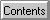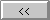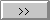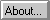# Introduction - Accuracy

Since the intended use of this program is Amateur Radio satellite tracking (or possibly naked-eye visual observation), great accuracy is not really necessary. Typical Amateur antenna systems (less than 20 dB gain) have beamwidths of 10 degrees or more. Thus, even a low-orbit satellite (at say 600 km altitude) need only be located to within 50 km or so!
There are 3 aspects to the accuracy of tracking predictions:
I. Numerical accuracy of calculations: This includes digital precision (loosely, rounding-off errors), uncertainty in parameter estimates, and the way in which parameters are incorporated into the model (i.e. some parameters may have more "leverage" to influence the results than others). For what it's worth, all calculations, including trig functions, in WinOrbit are done in "double precision" (about 14 significant figures). If realized completely, this could give an epoch-time precision of about +/- 100 microseconds, or a position precision of about +/-1 m for a typical orbital velocity. The bottom line here is that (unlike my old Z-80 system) with Windows/386/486-class machines, simple numerical error is the smallest of the effects on accuracy.

A more serious limitation is that all times are rounded to the nearest second by the Windows date conversion routine I used. Since a satellite in orbit has an average velocity of about 8 km/sec, this implies a possible (apparent) position error of up to 8 km at the nominal time, depending on the reference epoch time and the actual time of calculation. I'm still thinking about how to deal with this.
II. Orbital model accuracy: There are varying degrees of detail in representing the orbital motion of a satellite, depending on the required accuracy. WinOrbit incorporates 4 different user-selectable mathematical models, ranging from crude to moderately sophisticated. The effects of detail in the model appear in two places: in the exact position of the satellite as it orbits the earth; and in the way the orbit evolves with the passage of time. There is a tradeoff between complexity (and speed of calculation) and accuracy. A further tradeoff occurs because the published parameters (Keplerian Elements) for the models are adjusted to provide the best predictions for a particular model, and may not work as well for other models.
III. Observer model accuracy: Once the satellite's position is known to the desired degree of accuracy, we need to compute where to look (or point the antenna). Once again, there are levels of accuracy here, depending on the model. Accuracy here of better than 1 km is possible, depending upon how well the observer coordinates are known.
IV. Other non-idealities: The apparent position of a satellite may differ significantly from the true position, due to atmospheric distortion, especially at radio frequencies. See the related discussion of the Horizon.
Other aspects of accuracy relate to the presentation of the results:
The Maps: The accuracy of the on-screen display depends not only on the resolution of the display adaptor (how many independent pixels exist on the screen, like 1024x768) but also on the underlying map information. The maps are all generated by expanding, contracting, or distorting an internal bitmap which has a resolution of about 1000x500 pixels. Thus, accuracy better than about 0.3 degrees (about 20 miles at the equator) is impossible. In practice there are some problems with the bitmap, particularly in the antarctic regions, and near the International Date Line. Small islands have also been omitted in many cases. So don't expect too much.
Furthermore, numerical values are generally rounded off for printing or display, to reflect a reasonable level of accuracy (a percent or so). Just because the computer calculates 14 or so digits, doesn't mean they are all significant digits!

Note: WinOrbit calculates the satellite perigee, apogee, etc. based on the spherical earth model. Only the instantaneous satellite altitudes reflect the selected geodetic model (altitude above mean sea level).
Note : Doppler shifts are calculated based on the difference of two successive ranges, so the accuracy here will depend on the resolution interval as well as the above factors.
Note : The models used for antenna pattern, path loss, solar illumination, squint angle, and other parameters are described elsewhere.

Converted by Winhelp to Web